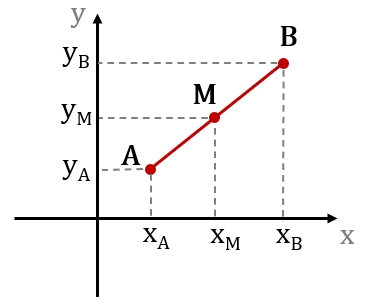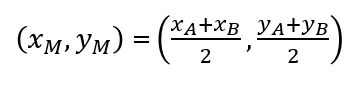# Midpoint Calculator

Use this calculator to determine the coordinates of the midpoint (M) of a line segment determined by its two end points (A, B). Find the midpoint of a segment AB.

,
,
Share calculator:

Embed this tool:
get code

## What is a midpoint?

In geometry, a midpoint is the point on a segment of a straight line which splits it in two equal halves which is why it is sometimes referred to as a halfway point. A segment is defined uniquely by two points (say A and B) and has a unique point (say M) which sits in its middle. A more technical way of describing a midpoint M is to say that it bisects the segment AB.

In a two-dimensional Cartesian coordinate plane each point has two coordinates - one on each axis. A simple visualization of the midpoint of a straight line AB and the corresponding coordinates is shown below:The point M splits the length of AB in two equal parts. Using a midpoint calculator one can find the coordinates of the midpoint by knowing the coordinates of the endpoints. Alternatively, if the coordinates of one endpoint and the midpoint are known, then the coordinates of the other point can be determined as well. See our endpoint calculator.

## Midpoint formula

The equation for finding the coordinates of the midpoint of a straight line AB defined by the points A and B is:where (xA, yA) are the coordinates of point A, (xB, yB) are the coordinates of point B, and (xA, xA) are the coordinates of M - the midpoint of AB as shown in the illustration above.

As we can see from the formula, the x-coordinate of the midpoint M of the line segment AB is the arithmetic mean of the x-coordinates of the two endpoints of the segment. Likewise, the y-coordinate of the midpoint is the mean of the y-coordinates of the endpoints. The formula is easy enough to apply even without the help of a calculator, but using a midpoint formula calculator certainly makes that a breeze.

## Midpoints in geometry

An example for using the midpoint equation can most easily be given in geometry. If one is given (or measured with a ruler) the coordinates of the two endpoints, one can determine the middle point. An example task would be:

What is the midpoint of the segment AB, if the coordinates of the first endpoint (A) are (2,6) and of the second (B) are (4, 18)?

To answer what the midpoint of AB is, simply replace the values in the formula to find the coordinates of the midpoint. In this case these are (2 + 4) / 2 = 3 and (6 + 18) / 2 = 12. So (xM, yM) = (3, 12) is the midpoint of the segment defined by A and B.

## Applications in physics

In physics, midpoint calculations have several prominent applications. For example, the center of mass of a given object is its center of gravity. In order to balance that object, support has to be provided to the midpoint to counteract gravity in such a way that neither end begins to dip. Finding the endpoint has obvious utility.

In tasks related to transportation or movement of objects in a straight line through two-dimensional space, the midpoint formula calculation can be useful in determining at what point or when a vehicle is halfway through to its destination. Obviously, objects rarely have the option of moving in a straight line for long distances, so such applications are mainly for teaching purposes.

#### Cite this calculator & page

If you'd like to cite this online calculator resource and information as provided on the page, you can use the following citation:
Georgiev G.Z., "Midpoint Calculator", [online] Available at: https://www.gigacalculator.com/calculators/midpoint-calculator.php URL [Accessed Date: 27 Mar, 2023].